### Home > CCG > Chapter Ch8 > Lesson 8.1.1 > Problem8-7

8-7.

Using the definition of polygon from the Math Notes box in this lesson determine whether each of the following figures is or is not a polygon. Justify your decisions for each figure.

1.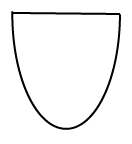Can polygons have curved lines?

No, it is not a polygon.

1.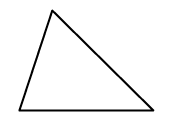Are polygons made up of straight line segments connected to each other?

1.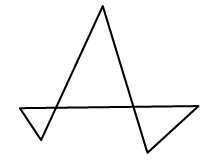Can the sides of polygons intersect?

No, it is not a polygon.

1.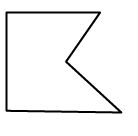See part (b) for help.

1.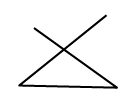Do all the line segments in a polygon have to connect?

No, it is not a polygon.

1.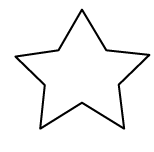Can the vertices of polygons also point inward?

Yes, this is called a non-convex polygon.

1.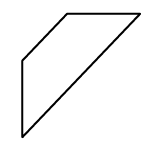See part (b) for help.

1.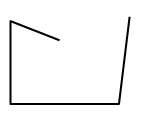See part (e) for help.